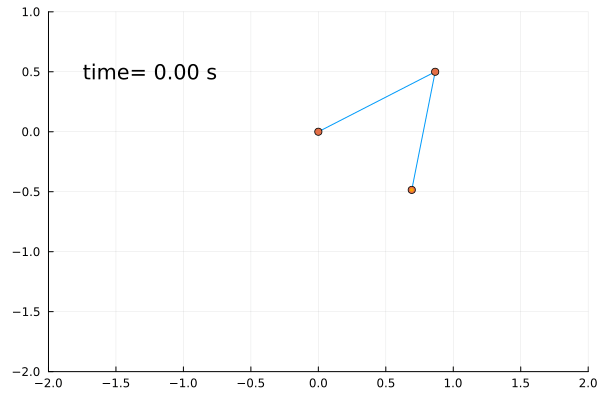# Double Pendulum ProblemThis animation illustrates the double pendulum problem.

Double pendulum formula translated from the matplotlib gallery.

using OrdinaryDiffEq

G = 9.8      # acceleration due to gravity, in m/s^2
L1 = 1.0     # length of pendulum 1 in m
L2 = 1.0     # length of pendulum 2 in m
L = L1 + L2  # maximal length of the combined pendulum
M1 = 1.0     # mass of pendulum 1 in kg
M2 = 1.0     # mass of pendulum 2 in kg
t_stop = 5   # how many seconds to simulate

function pendulum!(du, u, p, t)
(; M1, M2, L1, L2, G) = p

du = u

delta = u - u
den1 = (M1 + M2) * L1 - M2 * L1 * cos(delta) * cos(delta)
du = (
(
M2 * L1 * u * u * sin(delta) * cos(delta) +
M2 * G * sin(u) * cos(delta) +
M2 * L2 * u * u * sin(delta) - (M1 + M2) * G * sin(u)
) / den1
)

du = u

den2 = (L2 / L1) * den1
du = (
(
-M2 * L2 * u * u * sin(delta) * cos(delta) +
(M1 + M2) * G * sin(u) * cos(delta) -
(M1 + M2) * L1 * u * u * sin(delta) - (M1 + M2) * G * sin(u)
) / den2
)
nothing
end
pendulum! (generic function with 1 method)

th1 and th2 are the initial angles (degrees)

w10 and w20 are the initial angular velocities (degrees per second)

th1 = 120.0
w1 = 0.0
th2 = -10.0
w2 = 0.0

p = (; M1, M2, L1, L2, G)
prob = ODEProblem(pendulum!, deg2rad.([th1, w1, th2, w2]), (0.0, t_stop), p)
sol = solve(prob, Tsit5())

x1 = +L1 * sin.(sol[1, :])
y1 = -L1 * cos.(sol[1, :])

x2 = +L2 * sin.(sol[3, :]) + x1
y2 = -L2 * cos.(sol[3, :]) + y1

using Plots
gr()
anim = @animate for i in eachindex(x2)

x = [0, x1[i], x2[i]]
y = [0, y1[i], y2[i]]

plot(x, y, legend = false)
plot!(xlims = (-2, 2), xticks = -2:0.5:2)
plot!(ylims = (-2, 1), yticks = -2:0.5:1)
scatter!(x, y)

x = x2[1:i]
y = y2[1:i]

plot!(x, y, linecolor = :orange)
plot!(xlims = (-2, 2), xticks = -2:0.5:2)
plot!(ylims = (-2, 1), yticks = -2:0.5:1)
scatter!(
x,
y,
color = :orange,
markersize = 2,
markerstrokewidth = 0,
markerstrokecolor = :orange,
)
annotate!(-1.25, 0.5, "time= \$(rpad(round(sol.t[i]; digits=2),4,"0")) s")
end
gif(anim, fps = 10)